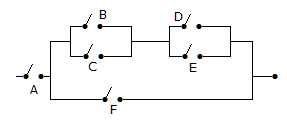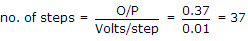# Electronics and Communication Engineering - Digital Electronics

21.

The Boolean expression for the circuit of the given figureA. A {F + (B + C) (D + E)} B. A [F + (B + C) (DE)] C. A + F + (B + C) (D + E)] D. A [F + (BC) (DE)]

Explanation:

B and C in parallel give B + C. Similarly D and E in parallel give D + E. (B + C) in series with (D + E) give (B + C) (D + E). Since F is in parallel we get F + (B + C) (D + E). Finally A is in series. Therefore we get A[F + (B + C) (D + E)].

22.

What will be BCD number when the output is 0.37 V?

 A. 00110111 B. 10110111 C. 11001000 D. 01001000

Explanation:BCD number is (00110111).

23.

The first machine cycle of an instruction is always

 A. a memory read cycle B. a fetch cycle C. a input/output read cycle D. a memory write cycle

Explanation:

Fetch cycle is always first machine cycle.

24.

A counter has N flip flops. The total number of states are

 A. N B. 2 N C. 2N D. 4 N

Explanation:

One flip-flop means 2 states and N flip-flops means 2N states.

25.

Out of S, R, J, K, Preset, Clear inputs to flip flops, the synchronous inputs are

 A. S, R, J, K only B. S, R, Preset, Clear only C. Preset, Clear only D. S, R only